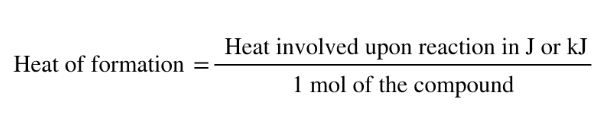# Problem: When 10.00 g of phosphorus is burned in O2(g) to form P4O10(s), enough heat is generated to raise the temperature of 2960 g of water from 18.0˚C to 38.0˚C. Calculate the heat of formation of P4O10(s) under these conditions.

###### FREE Expert Solution
• Determine how many moles of P4O10 produced using stoichiometry and then relate this to the total heat involved using the calorimetry equation
• Heat of formation is equal to the heat involved upon formation for every 1 mole of the compound• First thing is to setup the balanced equation where phosphorus (P4 (s)) reacts with oxygen gas (O(g)) forming P4O10 (s)
• Balanced equation will appear as:

P4 (s) + 5O2 (g) → P4O10 (s)

• Determine the # of moles of P4O10 starting with the mass of P4

MM of P4: P - 30.97 (4) = 123.88 g/mol

87% (240 ratings)###### Problem Details

When 10.00 g of phosphorus is burned in O2(g) to form P4O10(s), enough heat is generated to raise the temperature of 2960 g of water from 18.0˚C to 38.0˚C. Calculate the heat of formation of P4O10(s) under these conditions.